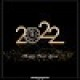# Inter maths 1b solutions

Inter maths 1B solutions for textbook are given.

These solutions are very easy to understand.

Study the text book lessons very well.

Observe the solutions and try them in your own methods.

# Intermediate mathematics 1b solutions

Inter Maths 1B solutions for textbook

Locus

Exercise 1(a)

Transformation of Axes

Exercise 2(a)

The Straight Line

Exercise 3(a)

Exercise 3(b)

Exercise 3(c)

Exercise 3(d)

Exercise 3(e)

Pair of Straight lines

Exercise 4(a)

Exercise 4(b)

Exercise 4(c)

Three Dimensional Coordinates

Exercise 5(b)

Direction Cosines and Direction Ratios

Exercise 6(a)

Exercise 6(b)

The Plane

Exercise 7(a)

Limits and Continuity

Exercise 8(a)

Exercise 8(b)

Exercise 8(c)

Exercise 8(d)

Exercise 8(e)

Differentiation

Exercise 9(a)

Exercise 9(b)

Exercise 9(c)

Exercise 9(d)

Applications of Derivaties

Exercise 10(a)

Exercise 10(b)

Exercise 10(c)

Exercise 10(d)

Exercise 10(e)

Exercise 10(f)

Exercise 10(g)

Exercise 10(h)

## Coordinate geometry and calculus solutions inter

Examination purpose solutions

1. Locus

Straight lines sa

Strainght lines la

Note : Observe the solutions and try them in your own methods.

You can also see

Inter Maths 1A textbook solutions

Inter Maths 1B textbook solutions

Inter Maths IIA textbook solutions

Inter Maths IIB textbook solutions

### 2 thoughts on “Inter maths 1b solutions”

1.There is no answer of 1B ch of 3,4

1.Answer 1b ch of 3 and 4